Start typing, then use the up and down arrows to select an option from the list.Jules Bruno
164views
8
Hey everyone. So here it says the following questions refer to the hydration curve given by law. So if we take a look at this situation curve we have, let's see on our Y axis. We have our ph volumes from 0 to 14 on our X axis, we have our milliliters of tightrope being added. We have points A, B, C and D. So there's quite a few things we can talk about in terms of this. But let's look at the questions and we'll see um what gets at it or what gets labeled. Alright, so here the titillation curve shows the tight rations of what so different combinations. If we look here, we need to locate what the equivalence point will be. Remember, the equivalence point can be determined by looking at what volume do we have? The sharpest rise within our ph we can see that happens around 30 mls where our sharpest rising peach happens here, midway through there is our equivalence points or equivalence point here is point C. And if we trace over here, we can see that point C has a ph of around nine or so, maybe a little bit higher than nine, but it's about nine. So think about what combination Between acid and base. Give me a page that's greater than seven at the equivalence point. So here, remember that happens when we have a weak acid being titrate, ID by a strong base. So that's the combination, weak acid strong base. That will give me a ph above seven for the equivalence point. So here we want a weak acid with a strong base would be the second option here, which point of the hydration curve represents a region where a buffer solution has formed. Remember a buffer exists between these two Before we get to the equivalence point. But remember for buffer to exist in some way to be generated, some of the titrate has to have been added at point A There is no tie trend that's been added yet, so there is no buffer that's been formed. All we have initially is weak acid. When we get to a point B. This represents our half equivalence point. This represents our ideal buffer. Remember at the half equivalent points where we have equal amounts of weak acid and its conjugate base. This is where we have our most ideal buffer. So, point B represents where a buffer has been formed, which point on the tire traction curve represents the equivalence point. We already discussed this, we said that was point C. And then finally, which of the following would be the best indicator to use in the tight rations. Remember an indicator can approximate what your equivalence point is by using the endpoint. That's the ph in which the indicator changes colors. So here we have our equivalence point around nine maybe slightly higher than nine. So we want to use an indicator that has uh basically a p K. That's close to that value. So, if we look here, we see that our last option here has a PK equal to nine, it's p K is going to be very close to the ph of the equivalence point. So that means it's testing for the endpoint and that endpoints around nine which is right next to the equivalence point, ph So this would be the best indicator to use. So again, the best indicator to use for a PhD attrition curve is the indicator that has a p k a closest to the equivalent point. Ph. So again, in this case, it will be our last option, which has a PK A equal to nine.1:259:00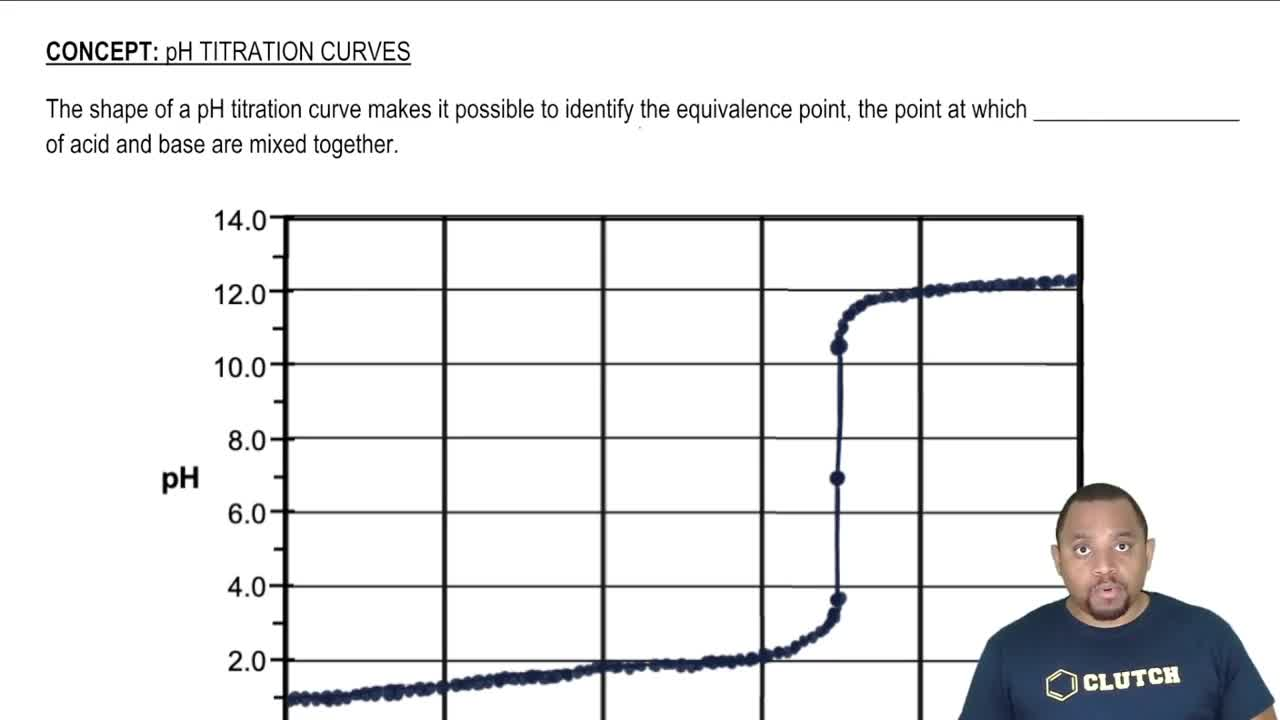04:13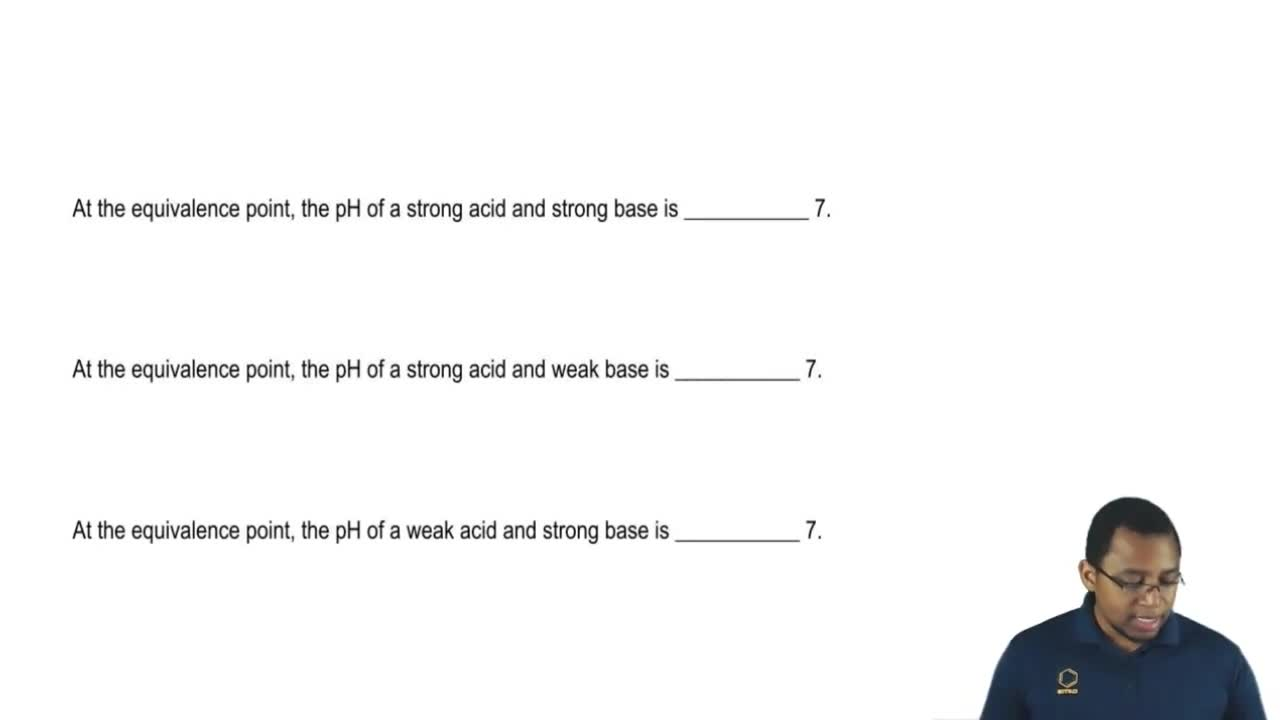01:39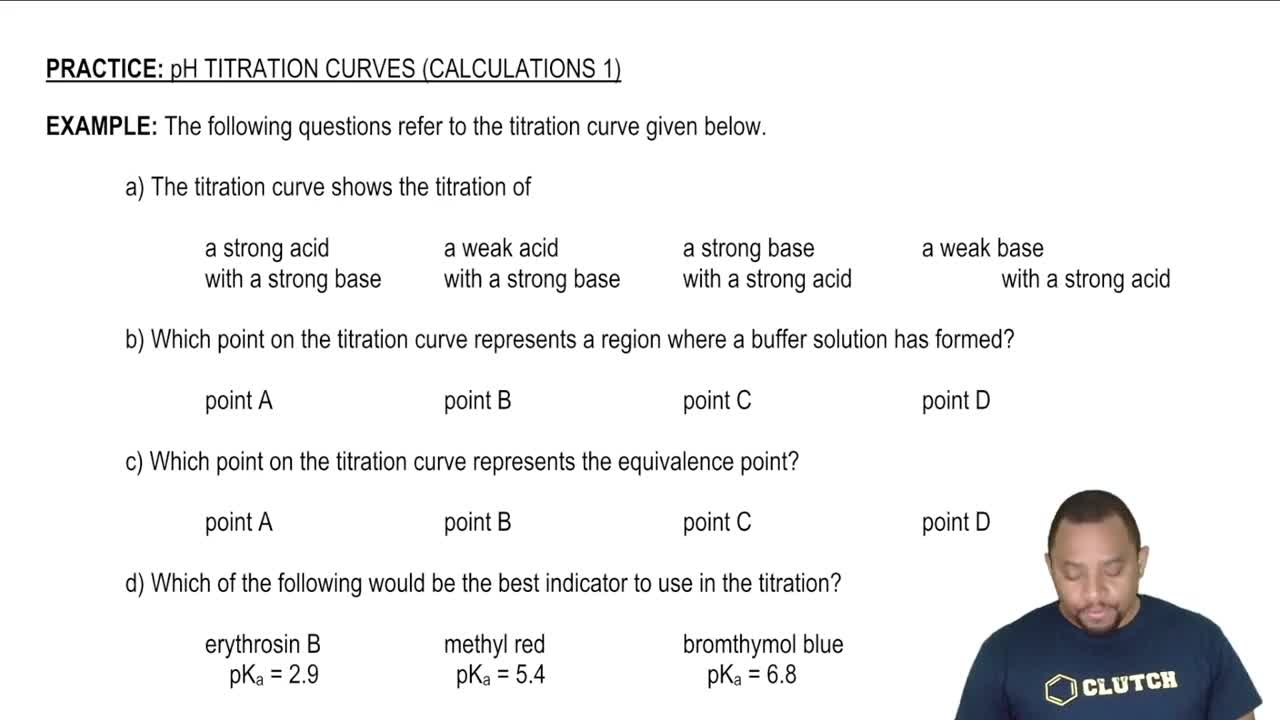06:43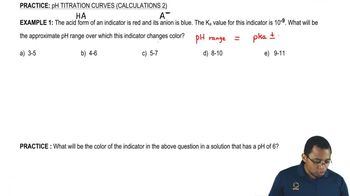03:06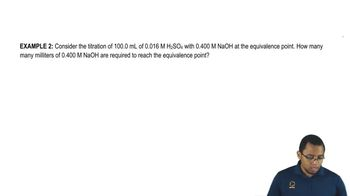06:2002:2701:27